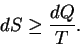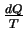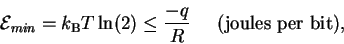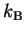Next: Bibliography Up: New Approaches in Mathematical Previous: Level 1. Machine Capacity:

# Level 2. The Second Law: Maxwell's Demon and the limits of computers

The Second Law of Thermodynamics can be expressed by the equation:(6)

(See https://alum.mit.edu/www/toms/ftp/secondlaw.ps for discussion of this equation.) The equation states that for a given amount of heat dQ entering a volume at some temperature T, the entropy will increase dS at least by.

We can relate entropy to the Shannon uncertainty if the probabilities describing the system states are the same for both functions, as is the case for molecular machines [13,4]. This connection and the constant temperature at which molecular machines operate at allow us to rewrite the Second Law in the following form:(7)

whereis Boltzmann's constant. This indicates that there is a relationship between the information R and the heat q. Remarkably, this same limit can be determined from the channel capacity (equation (5)) and the machine capacity. The interpretation of this equation is straightforward--there is a minimum amount of heat energy that must be dissipated (negative q) by a molecular machine in order for it to gain R = 1 bit of information.

Maxwell's Demon is a mythical creature who is supposedly able to open and close a tiny door between two containers of gas [14,15]. By observing the molecules that approach the door and by controlling the opening appropriately, the demon can allow the fast molecules through to one side and the slow ones to the other. Although any molecular biologist would expect the muscles and eyes of the demon to use energy, this is neglected by physicists. Also, they presume that the energy used to open the door can be regained when it is shut if it is attached to a spring. Such a demon could presumably create a macroscopic temperature difference between the two containers, and this could be used to run a heat engine. Apparently, the demon can supply energy merely by choosing between two alternatives. This would violate the Second Law of Thermodynamics.

Equation (7) applies to this problem. The demon always selects molecules in every scenario that he appears. We become duped by the story because the selective process is not explicitly stated as invoking the Second Law. But the Second Law always requires dissipation of heat energy to counterbalance selections made. Thus the demon is no longer a puzzle.

Equation (7) also applies both to molecular machines and to computers, so it sets a limit on computation. It is impossible to get temperatures of absolute zero because that would require infinite energy to remove all the heat energy. At any temperature above absolute zero, a computer must dissipate energy to make choices. As this energy must come from somewhere, we must feed the computer energy so that the computer can dissipate the energy while calculating our answers.

I thank Denise Rubens, Elaine Bucheimer and Paul N. Hengen for comments on the manuscript.Next: Bibliography Up: New Approaches in Mathematical Previous: Level 1. Machine Capacity:
Tom Schneider
2000-10-13